Courses

# Short Answers - Force and Laws of Motion, Science, Class 9 Class 9 Notes | EduRev

## Class 9 : Short Answers - Force and Laws of Motion, Science, Class 9 Class 9 Notes | EduRev

The document Short Answers - Force and Laws of Motion, Science, Class 9 Class 9 Notes | EduRev is a part of the Class 9 Course Class 9 Science by VP Classes.
All you need of Class 9 at this link: Class 9

Q1. State the difference in balanced and unbalanced force.
Ans.

 Balanced force Unbalanced force 1. Forces acting on a body from the opposite directions are same. 1. Forces acting on a body from two opposite directions are not same. 2. It does not change the state of rest or of motion of an object. 2. It do change the state of rest or of motion of an object.

Q2. What change will force bring in a body?
Ans.
Force can bring following changes in the body:
(i) It can change the speed of a body.
(ii) It can change the direction of motion of a body.
(iii) It can change the shape of the body.

Q3. When a motorcar makes a sharp turn at a high speed, we tend to get thrown to one side. Explain why?
Ans.
It is due to law of inertia. When we are sitting in car moving in straight line, we tend to continue in our straight-line motion. But when an unbalanced force is applied by the engine to change the direction of motion of the motorcar. We slip to one side of the seat due to the inertia of our body.

Q4. Explain why it is dangerous to jump out of a moving bus.
Ans.
While moving in a bus our body is in motion. On jumping out of a moving bus our feet touches the ground and come to rest. While the upper part of our body stays in motion and moves forward due to inertia of motion and hence we can fall in forward direction.
Hence, to avoid this we need to run forward in the direction of bus.

Q5. Why do fielders pull their hand gradually with the moving ball while holding a catch?
Ans.
While catching a fast moving cricket ball, a fielder on the ground gradually pulls his hands backwards with the moving ball. This is done so that the fielder increases the time during which the high velocity of the moving ball decreases to zero. Thus, the acceleration of the ball is decreased and therefore the impact of catching the fast moving ball is reduced.

Q6. In a high jump athletic event, why are athletes made to fall either on a cushioned bed or on a sand bed?
Ans.
In a high jump athletic event, athletes are made to fall either on a cushioned bed or on a sand bed so as to increase the time of the athlete’s fall to stop after making the jump. This decreases the rate of change of momentum and hence theforce.

Q7. How does a karate player breaks a slab of ice with a single blow?
Ans.
A karate player applies the blow with large velocity in a very short interval of time on the ice slab which therefore exerts large amount of force on it and suddenly breaks the ice slab.

Q8. What is law of conservation of momentum?
Ans.
Momentum of two bodies before collision is equal to the momentum after collision.
In an isolated system, the total momentum remain conserved.

Q9. Why are roads on mountains inclined inwards at turns?
Ans.
A vehicle moving on mountains is in the inertia of motion. At a sudden turn there is a tendency of vehicle to fall off the road due to sudden change in the line of motion hence the roads are inclined inwards so that the vehicle does not fall down the mountain.

Q10. For an athletic races why do athletes have a special posture with their right foot resting on a solid supporter?
Ans.
Athletes have to run the heats and they rest their foot on a solid supports before start so that during the start of the race the athlete pushes the support with lot of force and this support gives him equal and opposite push to start the race and get a good start to compete for the race.

Q11. Why do you think it is necessary to fasten your seat belts while travelling in your vehicle?
Or How are safety belts helpful in preventing any accidents?
Ans.
While we are travelling in a moving car, our body remains in the state of rest with respect to the seat. But when driver applies sudden breaks or stops the car our body tends to continue in the same state of motion because of its inertia. Therefore, this sudden break may cause injury to us by impact or collision. Hence, safety belt exerts a force on our body to make the forward motion slower.

Q12. Explain how momentum gets conserved in collision of two bodies.
Ans.
Consider two bodies i.e., balls A and B, the mass and initial velocities are mAuA and mBuB respectively before collision. The two bodies collide and force is exerted by each body. There is change in their velocities due to collision.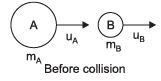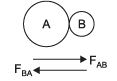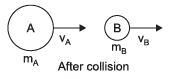The momentum of ball A before collision is mAuA and final momentum is mAvA.
The rate of change of momentum, during the collision for ‘A’ will be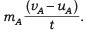Initial momentum of B is mBuB and final momentum is mBvB.
Rate of change of momentum of B will be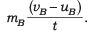According to the third law of motion, the force FAB exerted by ball A on ball B and the force FBA exerted by the ball B on ball A must be equal and opposite to each other.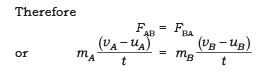∴ mAuA + mBuB = mAvA + mBvB
∴ (mAuA + mBuB) is the total momentum of the two balls A and B before collision and (mAvA + mBvB) is their total momentum after collision.
∴ The total momentum of the two balls remains unchanged or conserved provided no other external force acts.

Q13. When you kick a football it flies away but when you kick a stone you get hurt why?
Ans.
This is because stone is heavier than football and heavier objects offer larger inertia.
When we kick a football its mass is less and inertia is also less so force applied by our kick acts on it and hence it shows larger displacement but in case of stone, it has larger mass and offers larger inertia. When we kick (action) the stone it exerts an equal and opposite force (reaction) and hence it hurts the foot.

Q14. If a person jumps from a height on a concrete surface he gets hurt. Explain.
Ans.
When a person jumps from a height he is in state of inertia of motion. When he suddenly touches the ground he comes to rest in a very short time and hencethe force exerted by the hard concrete surface on his body is very high, and the person gets hurt.

Q15. What is the relation between Newton’s three laws of motion?
Ans
. Newton’s first law explains about the unbalanced force required to bring change in the position of the body.
Second law states/explains about the amount of force required to produce a given acceleration.
And Newton’s third law explains how these forces acting on a body are interrelated.

Q16. Give any three examples in daily life which are based on Newton’s third law of motion.
Ans.
Three examples based on Newton’s third law are :
1. Swimming: We push the water backward to move forward. action
– water is pushed behind reaction
– water pushes the swimmer ahead
2. Firing gun: A bullet fired from a gun and the gun recoils. action
– gun exerts force on the bullet reaction
– bullet exerts an equal and opposite force on the gun
3. Launching of rocket action
– hot gases from the rocket are released reaction
– the gases exert upward push to the rocket

Q17. A bullet of mass 20 g is horizontally fired with a velocity 150 m/s from a pistol of mass 2 kg. What is the recoil velocity of the pistol?
Ans.
Bullet Pistol m1 mass = 20 g = 0.02 kg m2 = 2 kg u1 = 0 u2 = 0 v1 = + 150 m/s v2 = ?
Total momentum of the pistol and bullet before firing, when the gun is at rest = m1u1 + m2u2 = (0.02 × 0) + (2 × 0)
= 0 kg m/s Total momentum of the pistol and bullet after it is fired = m1v1 + m2v2 = (0.02 kg × 150) + (2 kg × v) = 3 + 2v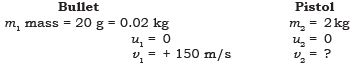∴ Total momentum after firing = Total momentum before firing 3 + 2v = 0
∴ v = 2 – 3 = – 1.5 m/s
Negative sign indicates that the direction in which the pistol would recoil is opposite to that of bullet.

Q18.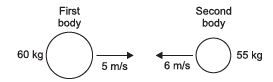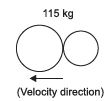Two bodies as shown in the figure collide with each other and join thereafter. With what velocity will they move after combining together?
Ans.
m1 = 60 kg
m2 = 55 kg
u1 = + 5 m/s
positive direction u2 = – 6 m/s negative direction v = ?
Total momentum of two bodies before collision = m1u1 + m2u2 = 60 × 5 + 55 × (– 6)
= – 30 kg m/s If v is the velocity of two joined bodies.
After collision, the total momentum will be = m1v1 + m2v2 (∴ v1 = v2) = (m1 + m2)
v = (60 + 55) kg × v m/s = 115 v
∴ According to law of conservation of momentum m1u1 + m2u2 = m1v1 + m2v2 – 30 kg m/s = 115 v
∴  v = 115 –30 = – 0.26 m/s
∴ Two bodies will move with velocity 0.26 m/s in the direction of the second body.

Q19. The surface gravity of the Sun is 274 m/s2. How many Earth’s g is this?
Ans.
g = 9.80 m/s2, gSun = 274 m/s2, 274/9.8 = 27.96 times of Earth gravity

Q20. A parked car is pushed with a force of 342 N from rest. How far does the car travel in 12 seconds? Its mass is 989 kg. How far does the car travel now if the pushing force is doubled?
Ans.
F = 342N, vo = 0, x = ?, t = 12s, m = 989 kg,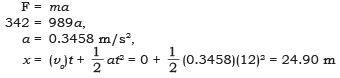If the pushing force is doubled then the acceleration is doubled. Because the relationship between x and a is linear, if the acceleration is doubled then the distance is also doubled. Therefore, the car will travel 49.80 m.

Q21. A 1027 kg truck is resting at a stop light. The truck moves with a force of 1528 N for 22 s. Then the truck travels at a constant velocity for 10 seconds. Finally, the truck stops with a force of 4056 N. Calculate the distance travelled by truck during this journey.
Ans.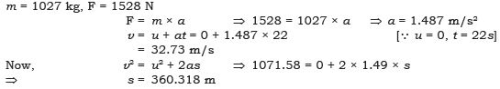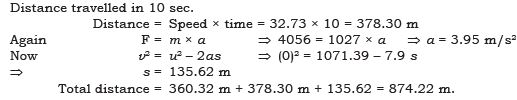Q22. Skydivers experience terminal speed. (a) State what terminal speed is and how it occurs.
(b) Explain the effect opening a parachute has on the skydiver’s terminal speed
Ans.
(a) The speed at which a falling object’s weight is cancelled by air resistance is called terminal speed.
(b) Air friction increases with speed. Once the force of friction equals weight, the net force on the skydiver is zero.

Q23. Two students are standing on the roller skates and pulling on a rope:
(a) The student on the left is pulling with 432 N. State how much force the student on the right is pulling with.
(b) The student on the left and his boat have a total mass of 103 kg, and the friction of the land is 98.1 N. Calculate the acceleration of the student on the left.
Ans.
(a) 432 N.
(b) Net force on the student is 432 – 98.1 = 333.9N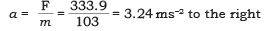Q24. A big luggage bag was thrown from an aircraft on the ground.
(a) State the magnitude of the acceleration of the bag as soon as it is dropped.
(b) After falling for some time, the bags acceleration is zero, but it is still falling. Explain, using net forces, how and why this happened.
Ans.
(a) 9.8 ms–2.
(b) Air resistance increases with speed. Eventually the air resistance equals weight, so the net force is zero (hence acceleration is zero).

Q25. If a bird feather and a feather made of metal are dropped on earth, the bird feather takes much longer to reach the ground. Explain why the bird feather falls slower than the feather made of metal.
Ans.
The metal feather has more inertia, so the force of air resistance has less effect. The metal feather has more weight, so its net force is greater.

Q26. (a) Explain how much force is required to stop an object with the force then to start it moving.
(b) State the effect friction would have on these forces.
Ans.
(a) It is the same. The inertia of an object is the same no matter what its speed is.
(b) Friction makes it easier to slow things down as it is a force which opposes motion.

Q27. If a trolley is initially at rest it takes a lot of force to get it moving and then a much smaller consistent force to keep it moving at constant speed.
(a) If the force of friction acting on a moving trolley is 15.42 N, state the force required to maintain a constant velocity.
(b) State the net force on the trolley at constant velocity.
(c) If the trolley is accelerated to a faster speed and then maintain its motion at that speed. Explain the effect this would have on the force required to maintain constant speed.
Ans.
(a) 15.42 N
(b) 0 N
(c) Greater, since the trolley is moving faster and friction is proportional to speed.

Q28. In a frictionless environment/vacuum, two objects with different masses will experience different forces due to gravity but the same acceleration. Explain.
Ans.
The force due to gravity (weight) is proportional to mass (W = mg), so heavier objects have more force due to gravity. The acceleration is inversely proportional to mass a = F/m, so heavier objects need more force to experience the same acceleration (they have more inertia).

Q29. If you are in a plane travelling at 600 km/h and a hand bag falls from an overhead compartment:
(a) Explain why the hand bag doesn’t go towards the back of the plane.
(b) State the hand bags horizontal speed relative to the ground.
(c) State the hand bags horizontal speed relative to you.
Ans.
(a) No force is acting on it which would change its speed, so it continues at the speed of the plane.
(b) 600 km/h.
(c) 0 km/h.

Q30. When you are weighing yourself standing next to the table. If you push yourself down on the table , the scales read less, and if you pull the table, the scales read more. Explain, using Newton’s third law.
Ans.
When you push down on the table, the table pushes you upward. This tends to lift you off the scales and decrease the reading on the scale. If you pull the table , it will push you downwards and increase the reading.

Q31. If somebody weighs 500N on Earth, calculate how much the same person weighs on Planet X, where the acceleration of gravity is 26ms–2.
Ans.
The person’s mass is 500 ÷ 9.8 = 51 kg,
So his weight on Planet X is 51 × 26 = 1326 N.

Offer running on EduRev: Apply code STAYHOME200 to get INR 200 off on our premium plan EduRev Infinity!

75 docs|14 tests

,

,

,

,

,

,

,

,

,

,

,

,

,

,

,

,

,

,

,

,

,

,

,

,

,

,

,

;